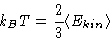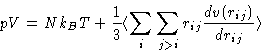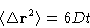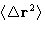# What can we get form the NVE MD simulations?

Temperature:
As the MD simulations are performed in the microcanonical ensemble, temperature is not set, it is obtained from the simulations. For the three-dimensional case:(1)
Pressure:
Same way as in the MC using the virial formula.(2)
Structural Information:
Can also be calculated exactly as in the Monte Carlo simulations. Exactly same code for g(r) can be used.
Diffusion coefficient:
This is what cannot be obtained from Monte Carlo. The easiest way to calculate it from MD is by Einstein relation:(3)
The trajectory, obtained in the MD run, givesas a function of t. It is plotted, and the tangent of the linear fit gives diffusion coefficient. The algorithm:
1.
Configurations (snapshots) are saved every n steps during the MD run
2.
The whole system trajectory is read by a separate program into the arrayx(k,ishot,ipart) that stores k-th (x and y) coordinate of the particle ipart at the ishot's frame saved.
3.
The mean squared displacement is averaged over the particles and over the initial configurations (see Code Example (8)).

Time-correlation functions and spectra:
Calculated exactly like diffusion coefficient. We average over the particles and initial conditions The Fourier transforms of different time correlation functions are compared with spectroscopic experiments:
• Dipole correlations are relevant for the Dielectric and Infra-red or Raman spectroscopy
• Orientation correlations of the C-H bonds can be measured by the proton NMR
• etc.Next: Fortran Code Up: Molecular Dynamics II Previous: Molecular Dynamics II

© 1997 Boris Veytsman and Michael Kotelyanskii
Wed Nov 26 01:51:43 EST 1997